# Comparing growth models

library(biogrowth)
library(tidyverse)

One of the main goals of biogrowth is to ease comparison between different modeling approaches. For that reason, the package includes several growth models, as well as the possibility to fix any model parameters. With this same goal, the package also includes the function compare_growth_fits() to ease the comparison between model fits, both using graphical representations and statistical indexes. The type of information returned by this function varies slightly depending on the type of model. For that reason, this vignette is divided in subsections for each type.

## Models fitted under constant environmental conditions

For this demonstration, we will use the data on the growth of Salmonella spp. included in the package.

data("growth_salmonella")
#> # A tibble: 6 × 2
#>    time  logN
#>   <dbl> <dbl>
#> 1  0     3.36
#> 2  1.95  3.4
#> 3  2.78  3.44
#> 4  3.78  3.31
#> 5  4.8   3.39
#> 6  5.7   3.65

This data comprises data gathered under constant environmental conditions. Therefore, we will describe it using only primary models (environment="constant" in fit_growth()). We will compare three modeling approaches. The first one is the Baranyi model:

fit1 <- fit_growth(growth_salmonella,
list(primary = "Baranyi"),
start = c(lambda = 0, logNmax = 8, mu = .1, logN0 = 2),
known = c(),
environment = "constant"
)

The second one is the Baranyi model fixing the duration of the lag phase to zero (lambda=0):

fit2 <- fit_growth(growth_salmonella,
list(primary = "Baranyi"),
start = c(logNmax = 8, mu = .1, logN0 = 2),
known = c(lambda = 0),
environment = "constant"
)

And the third one is the modified Gompertz model:

fit3 <- fit_growth(growth_salmonella,
list(primary = "modGompertz"),
start = c(C = 8, mu = .1, logN0 = 2, lambda = 0),
known = c(),
environment = "constant"
)

Once the three models have been fitted, we can call compare_growth_fits(). This function takes as only argument a list of models. Although it does not have to be named, we recommend naming it because every output will be labeled according to these names.

my_models <- list(
Baranyi = fit1,
Baranyi no-lag = fit2,
mod Gompertz = fit3
)

salmonella_models <- compare_growth_fits(my_models)

The function return an instance of GrowthComparison with several S3 methods for comparing among the models fitted. The print method shows the type of model fitted, as well as several statistical indexes describing the goodness of fit. The models are arranged in descending AIC, so the first row would included the preferred model.

print(salmonella_models)
#> Comparison between models fitted to data under isothermal conditions
#>
#> Statistical indexes arranged by AIC:
#>
#> # A tibble: 3 × 5
#>   model             AIC    df           ME   RMSE
#>   <chr>           <dbl> <int>        <dbl>  <dbl>
#> 1 Baranyi        -29.7     17  0.000000401 0.0919
#> 2 mod Gompertz   -18.7     17  0.000000395 0.119
#> 3 Baranyi no-lag   4.49    18 -0.000000513 0.224

GrowthComparison also includes an S3 plot method to evaluate the model fits. It can make three type of plots. The default one is a comparison between the fitted curves and the experimental data. Note that the model names in the legend are the sames as those defined in the input argument.

plot(salmonella_models)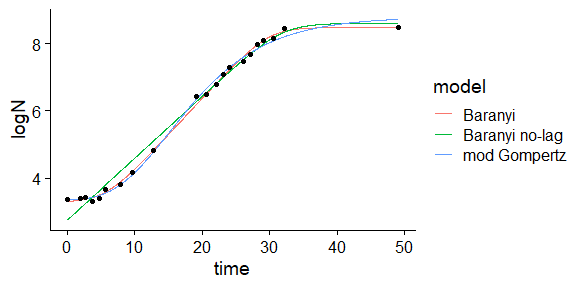By passing, type=2 to the plot function, the function instead compares the parameter estimates. In this plot, the dots show the estimated values and the error bars their associated standard errors. Note that different models have different model parameters (e.g. the modified Gompertz uses $$C$$ instead of $$logN_{max}$$), so some bars may be missing in some facets. The same happens if some model parameter was fixed prior to model fitting.

plot(salmonella_models, type = 2) +
theme(axis.text.x = element_text(angle = 45, hjust = 1))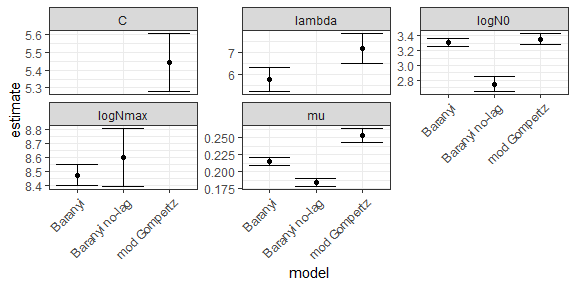The last type of plot is a plot of the residuals. This plot includes a trend line of the residuals (calculated using loess). Clear deviations of this trend line with respect to the horizontal dashed line (residual = 0) is often an indicator of a poor model fit.

plot(salmonella_models, type = 3)
#> geom_smooth() using formula = 'y ~ x'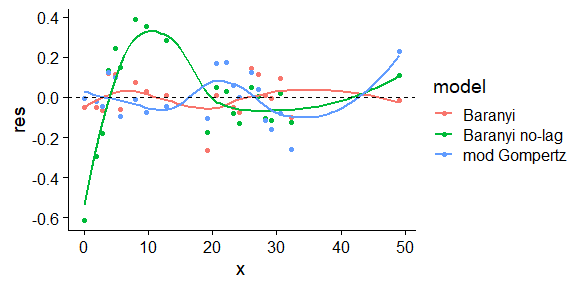The statistical indexes of the fit can be retrieved using the summary()

summary(salmonella_models)
#> # A tibble: 3 × 5
#>   model             AIC    df           ME   RMSE
#>   <chr>           <dbl> <int>        <dbl>  <dbl>
#> 1 Baranyi        -29.7     17  0.000000401 0.0919
#> 2 mod Gompertz   -18.7     17  0.000000395 0.119
#> 3 Baranyi no-lag   4.49    18 -0.000000513 0.224

And the table of parameter estimates using coef()

coef(salmonella_models)
#> # A tibble: 11 × 4
#>    model          parameter estimate std.err
#>    <chr>          <chr>        <dbl>   <dbl>
#>  1 Baranyi        lambda       5.77  0.561
#>  2 Baranyi        logNmax      8.47  0.0752
#>  3 Baranyi        mu           0.215 0.00545
#>  4 Baranyi        logN0        3.31  0.0574
#>  5 Baranyi no-lag logNmax      8.60  0.209
#>  6 Baranyi no-lag mu           0.184 0.00604
#>  7 Baranyi no-lag logN0        2.75  0.103
#>  8 mod Gompertz   C            5.44  0.164
#>  9 mod Gompertz   mu           0.253 0.0101
#> 10 mod Gompertz   logN0        3.35  0.0743
#> 11 mod Gompertz   lambda       7.17  0.676

## Models fitted under dynamic environmental conditions

In the case of models fitted under dynamic environmental conditions, the input arguments, output and S3 methods are identical to the ones for static environmental conditions. Please check the documentation of compare_growth_fits() for an example.

## Growth models fitted to multiple experiments (global fit)

In the case of global fits, the compare_growth_fits() function has many similarities with respect to fittings using approach="single". We first need to fit (at least) two growth models to a set of experiments:

data("multiple_counts")
data("multiple_conditions")

sec_models <- list(temperature = "CPM", pH = "CPM")

known_pars <- list(Nmax = 1e8, N0 = 1e0, Q0 = 1e-3,
temperature_n = 2, temperature_xmin = 20,
temperature_xmax = 35,
pH_n = 2, pH_xmin = 5.5, pH_xmax = 7.5, pH_xopt = 6.5,
temperature_xopt = 30)

my_start <- list(mu_opt = .8)

global_fit <- fit_growth(multiple_counts,
sec_models,
my_start,
known_pars,
environment = "dynamic",
algorithm = "regression",
approach = "global",
env_conditions = multiple_conditions
)

sec_models <- list(temperature = "CPM", pH = "CPM")

known_pars <- list(Nmax = 1e8, N0 = 1e0, Q0 = 1e-3,
temperature_n = 1, temperature_xmin = 20,
temperature_xmax = 35,
pH_n = 2, pH_xmin = 5.5, pH_xmax = 7.5, pH_xopt = 6.5,
temperature_xopt = 30)

my_start <- list(mu_opt = .8)

global_fit2 <- fit_growth(multiple_counts,
sec_models,
my_start,
known_pars,
environment = "dynamic",
algorithm = "regression",
approach = "global",
env_conditions = multiple_conditions
) 

Then, we can call the function passing a (named) list with the models as only argument:

global_comparison <- compare_growth_fits(list(n=2 = global_fit, n=1 = global_fit2))

This returns and instance of GlobalGrowthComparison. This function has the same S3 methods as GrowthComparison. The only difference is that the comparison of the growth curves is divided by facets for each experiment

plot(global_comparison)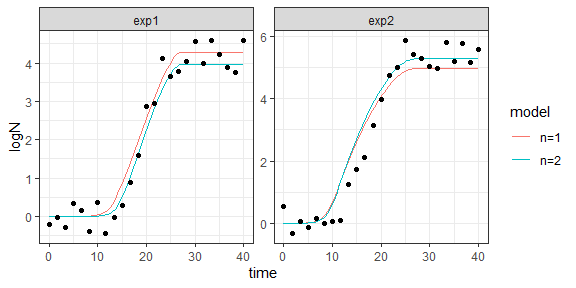The same applies to the residuals plot

plot(global_comparison, type = 3)
#> geom_smooth() using formula = 'y ~ x'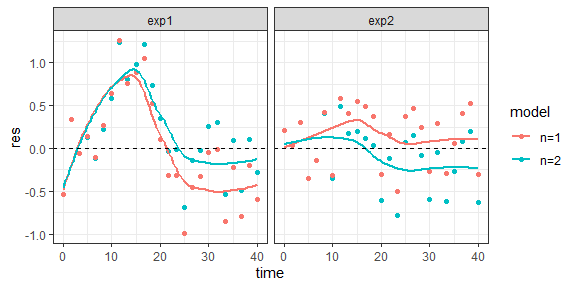The rest, are exactly the same

print(global_comparison)
#> Comparison between models fitted using a global approach
#>
#> Statistical indexes arranged by AIC:
#>
#> # A tibble: 2 × 5
#>   model   AIC    df     ME  RMSE
#>   <chr> <dbl> <int>  <dbl> <dbl>
#> 1 n=2    66.0    49 0.0460 0.458
#> 2 n=1    71.8    49 0.0715 0.486
plot(global_comparison, type = 2)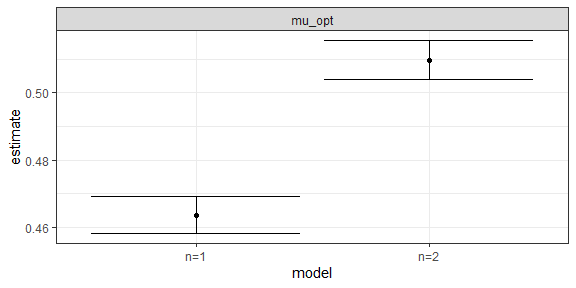summary(global_comparison)
#> # A tibble: 2 × 5
#>   model   AIC    df     ME  RMSE
#>   <chr> <dbl> <int>  <dbl> <dbl>
#> 1 n=2    66.0    49 0.0460 0.458
#> 2 n=1    71.8    49 0.0715 0.486
coef(global_comparison)
#> # A tibble: 2 × 4
#>   model parameter estimate std.err
#>   <chr> <chr>        <dbl>   <dbl>
#> 1 n=2   mu_opt       0.510 0.00581
#> 2 n=1   mu_opt       0.464 0.00559

## Fitting of secondary models

For comparing the fit of secondary growth models, the package includes the compare_secondary_fits() function. The approach of this function is very similar to the one implemented in compare_growth_fits(). As an illustration, we will use the example data included in the package.

data("example_cardinal")
#>   temperature pH           mu
#> 1    0.000000  5 9.768505e-04
#> 2    5.714286  5 2.624919e-03
#> 3   11.428571  5 0.000000e+00
#> 4   17.142857  5 1.530706e-04
#> 5   22.857143  5 2.301817e-05
#> 6   28.571429  5 3.895598e-04

We first need to fit at least two different models to this dataset. In this case, we will compare the effect of using $$n=1$$ or $$n=2$$ in the secondary model for temperature.

sec_model_names <- c(temperature = "Zwietering", pH = "CPM")

known_pars <- list(mu_opt = 1.2, temperature_n = 1,
pH_n = 2, pH_xmax = 6.8, pH_xmin = 5.2)

my_start <- list(temperature_xmin = 5, temperature_xopt = 35,
pH_xopt = 6.5)

fit1 <- fit_secondary_growth(example_cardinal, my_start, known_pars, sec_model_names)

known_pars <- list(mu_opt = 1.2, temperature_n = 2,
pH_n = 2, pH_xmax = 6.8, pH_xmin = 5.2)

fit2 <- fit_secondary_growth(example_cardinal, my_start, known_pars, sec_model_names)

We can now pass both models to compare_secondary_fits() as a named list

my_models <- list(n=1 = fit1, n=2 = fit2)

secondary_comparison <- compare_secondary_fits(my_models)

The function returns an instance of SecondaryGrowthComparison. It includes similar S3 methods as those defined for the other types of model fits. The main difference is that it cannot plot directly the fitted model against the data. The reason for that is that fit_secondary_growth can use an arbitrary number of dimensions (in the example: temperature and pH). Therefore, representing the predictions as a function of a unique factor is not very informative.

print(secondary_comparison)
#> Comparison between secondary growth models fitted to a dataset of growth rates
#>
#> Statistical indexes arranged by AIC:
#>
#> # A tibble: 2 × 5
#>   model   AIC    df       ME   RMSE
#>   <chr> <dbl> <int>    <dbl>  <dbl>
#> 1 n=1   -226.    61 -0.00641 0.0393
#> 2 n=2   -122.    61 -0.0501  0.0884

plot(secondary_comparison)
#> geom_smooth() using formula = 'y ~ x'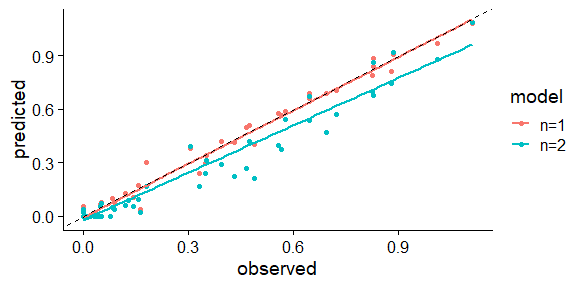plot(secondary_comparison, type = 2)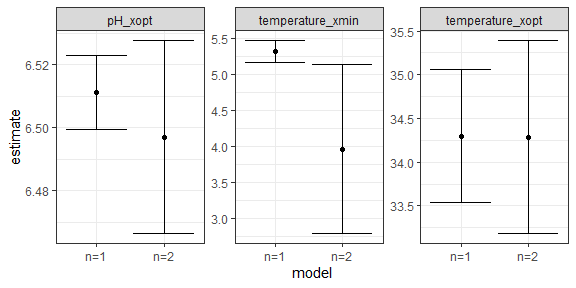coef(secondary_comparison)
#> # A tibble: 6 × 4
#>   model parameter        estimate std.err
#>   <chr> <chr>               <dbl>   <dbl>
#> 1 n=1   temperature_xmin     5.32  0.150
#> 2 n=1   temperature_xopt    34.3   0.763
#> 3 n=1   pH_xopt              6.51  0.0117
#> 4 n=2   temperature_xmin     3.96  1.17
#> 5 n=2   temperature_xopt    34.3   1.10
#> 6 n=2   pH_xopt              6.50  0.0306
summary(secondary_comparison)
#> # A tibble: 2 × 5
#>   model   AIC    df       ME   RMSE
#>   <chr> <dbl> <int>    <dbl>  <dbl>
#> 1 n=1   -226.    61 -0.00641 0.0393
#> 2 n=2   -122.    61 -0.0501  0.0884